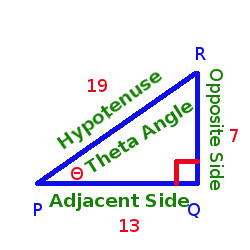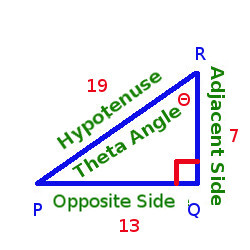Arithmetic
Arithmetic Progression
Associative Property
Averages
Brackets
Closure Property
Commutative Property
Conversion of Measurement Units
Cube Root
Decimal
Divisibility Principles
Equality
Exponents
Factors
Fractions
Fundamental Operations
H.C.F / G.C.D
Integers
L.C.M
Multiples
Multiplicative Identity
Multiplicative Inverse
Numbers
Percentages
Profit and Loss
Ratio and Proportion
Simple Interest
Square Root
Unitary Method
Algebra
Cartesian System
Order Relation
Polynomials
Probability
Standard Identities & their applications
Transpose
Geometry
Basic Geometrical Terms
Circle
Curves
Angles
Define Line, Line Segment and Rays
Non-Collinear Points
Parallelogram
Rectangle
Rhombus
Square
Three dimensional object
Trapezium
Triangle
Trigonometry
Trigonometry Ratios
Data-Handling
Arithmetic Mean
Frequency Distribution Table
Graphs
Median
Mode
Range

Videos
Solved Problems
Home >> Trigonometry Ratios >> Examples

## Find ratio of Sin, Cosec, Cos, Sec, Tan and Cot - Trigonometry : Solved Examples

 T Ratios of Angles - 30, 45, 60 & 90 degree angles Find Height, Distance using T - Ratios

 △ PQR is right angle triangle at Q, PQ=13, QR=7, PR=19. Write Cosec R, Sec P, Cot P Lets draw the right angle triangle with the above mentioned details Given thing are Right angle at Q Theta Angle at P (As mentioned in statement Sec P, Cot P that means angle P should be taken as Theta)Hypotenuse : PR = 19 Opposite Side : QR = 7 (Opposite to ∠ Θ) Adjacent Side : PQ = 13 (Adjacent to ∠ Θ) Cot P = Adjacent Side / Opposite Side = 13/7 Sec P = Hypotenuse / Adjacent Side = 19/13 For Cosec the Theta Angle is at R (As mentioned in statement "Cosec R" that means angle R should be taken as Theta) As Cosec angle is now R, the adjacent side and opposite side of the triangle will be as belowYou will see that Theta angle is now at R, So Hypotenuse : PR = 19 Opposite Side : PQ = 13 (Opposite to ∠ Θ) Adjacent Side : RQ = 7 (Adjacent to ∠ Θ) So, Cosec R = Hypotenuse / Opposite Side = 19/13

### Related Question Examples

 △ ABC is right angle triangle at B, AB=8, BC=11, AC=13. Write sin C, cos A, tan C △ PQR is right angle triangle at P, PQ=9, QR=11, RP=7. Write sin Q, cos R, tan Q △ ABC is right angle triangle at A, AB=11, BC=13, AC=8. Write sin A, cos C, tan A △ PQR is right angle triangle at Q, PQ=7, QR=9, PR=11. Write sin R, cos R, tan P △ ABC is right angle triangle at B, AB=11, BC=9, AC=17. Write sin A, cos C, tan C △ PQR is right angle triangle at Q, PQ=13, QR=7, PR=19. Write sin R, cos P, tan P If cos Θ = 11/13, find sin Θ and tan Θ If sin Θ = 7/11, find cos Θ and tan Θ If cos Theta = 2/3, find sin and tan theta If Tan Θ = 3/5, find sin Θ and cos Θ △ ABC is right angle triangle at B, AB=8, BC=11, AC=13. Write Cosec C, Sec A, Cot C If Sin Θ = 5/7, find cos Θ and tan Θ If Tan Θ = 2/3, find Sin Θ and Cos Θ △ ABC is right angle triangle at A, AB=11, BC=13, AC=8. Write Cosec A, Sec C, Cot A △ PQR is right angle triangle at P, PQ=9, QR=11, RP=7. Write Cosec Q, Sec R, Cot Q △ PQR is right angle triangle at Q, PQ=7, QR=9, PR=11. Write Cosec R, Sec R, Cot P △ ABC is right angle triangle at B, AB=11, BC=9, AC=17. Write Cosec A, Sec C, Cot C △ PQR is right angle triangle at Q, PQ=13, QR=7, PR=19. Write Cosec R, Sec P, Cot P If Sec Θ = 11/13, find Cosec Θ and Cot Θ If Cosec Θ = 7/11, find Sec Θ and Cot Θ If Cot Θ = 3/5, find Cosec Θ and Sec Θ If Sec Θ = 2/3, find Cosec Θ and Cot Θ If Cosec Θ = 5/7, find Sec Θ and Cot Θ If Cot Θ = 2/3, find Cosec Θ and Sec Θ

Copyright@2022 Algebraden.com (Math, Algebra & Geometry tutorials for school and home education)